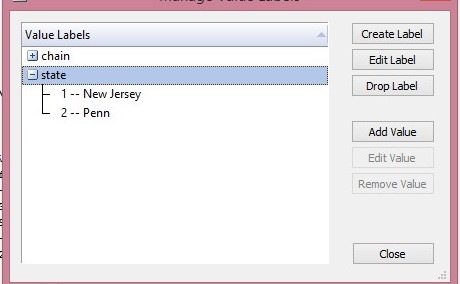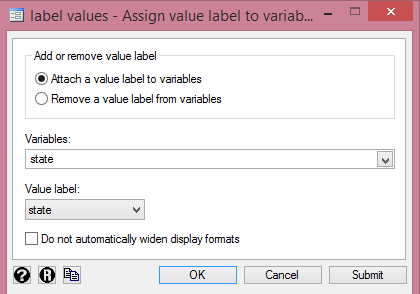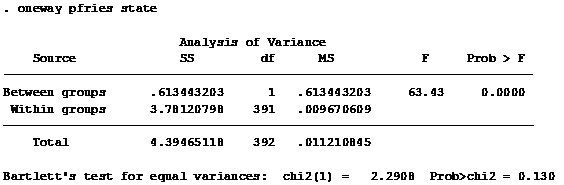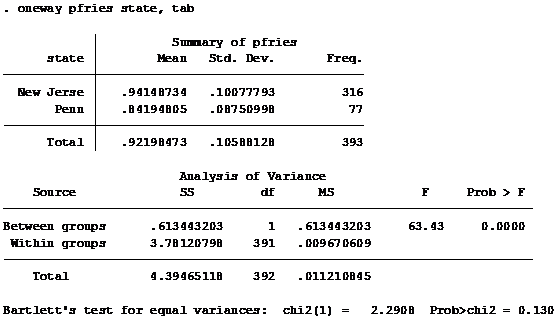## One-way ANOVA Procedure using Stata

Preamble
Ever wondered what the buzz about ANOVA is all about? ANOVA simply means analysis of variance. It is a statistical method in which the variation in a set of observations is divided into distinct components. It is an extension of the t and z test developed by Roland Fisher. The ANOVA procedure is of two types – one-way and two-way- with several dimensions. But for this tutorial, only the one-way ANOVA will be discussed while the two-way procedure will be covered in subsequent lectures.

Why is ANOVA useful in data analysis?
One importance of carrying out ANOVA is to determine if the average value (that is, the mean) of a dependent variable (the regressand, outcome variable, and endogenous variable) is the same in two or more unrelated, independent groups. Thus, the one-way ANOVA indicates whether the mean of a dependent variable is the same or differs across independent unrelated groups. The moment you understand how to compute ANOVA and interpret your table, you will always want to incorporate it in your study or research…that is, subject to data meeting some salient conditions.

Practically, ANOVA can be used to measure the patterns of individuals, environments, disciplines etc. across groups. For instance, you can use a one-way ANOVA to determine whether weight loss differs based on diet programs among women (i.e., your dependent variable would be "weight loss", measured from 65-80kg, and your explanatory variable would be "weight loss programmes ", which are in three groups: "keto plan", "plant-based plan, and "vegetarian plan"). Alternately, a one-way ANOVA could be used to understand whether there is a difference in insurance schemes based on professions (i.e., your dependent variable would be "insurance" and your independent variable would be "profession", which has four categories: "mining", "teaching", "oil drilling", "lab scientist").

Thus, when the difference between the groups is statistically significant, it is possible to determine which specific groups are significantly different from each other using post estimation tests. These tests are necessary because the one-way ANOVA only says that at least two groups are different without giving information as to which specific groups were significantly different from each other.
Given this preamble, here is a “step-by-step” tutorial showing you how to carry out ANOVA and post-estimation checks using Stata analytical package. But before I proceed, it is important for you to understand some basic rules underlying the use of one-way ANOVA procedure. That is, your data must meet these criteria failing which your results may be invalidated if they are not adhered to. There are six (6) of them:

Rules:
These six "rules" represent the blueprint guiding the use of the one-way ANOVA technique. If any is not satisfied, you may obtain invalid results. Please note that the first three assumptions are closely related to the nature of your data and study structure (that is, directly related to your choice of variables), thus Stata cannot validate those while the last three must be met using some Stata criterion. It is therefore important that you ascertain that your study meets these conditions before proceeding with the one-way ANOVA.

· Rule #1: Make sure that the dependent variable (regressand, outcome variable) is cardinal and measured in continuous terms. Some example of variables in measured in continuous terms are: distance (measured in miles, kilometres), weight (measured in stone, pounds, kilogramme, and grams); wages (measure in local currency) and so on. These are called continuous variables. In the event that you have ordinal variables, then consider doing a Kruskal-Wallis H test.

· Rule #2: The explanatory variable (regressor, independent variable) ought to comprise two or more categorical, independent (unrelated) groups. Some examples of these categorical variables are income group (3 groups: high-income, middle income and low income); grade (4 groups: excellent, very good, good, and poor); demography (2 groups: rural and urban); banking (3 groups: investment, mortgage, microfinance) etc. So make sure that your explanatory variable is a categorical variable.

· Rule #3: Ensure that you have independence of observations. That is, your observations must not over-lap across the different groups. This simply means that there must be no relationship between the observations in each group or between the groups themselves. For instance, an observation in a “high-income” group must not be represented again in a “low-income” group. Needless to say that, participants across the groups must be different. But where an exception is the case, the repeated measures of ANOVA should be used rather than the one-way ANOVA.

· Rule #4: Be wary of outliers. These are figures that are either abnormally high or low, that is, they do not follow the typical pattern in a particular variable. The presence of outliers can bias your results. However, they can easily be tested in Stata by using the Boxplot or summary syntax (sum for short). The syntax computes the mean, standard deviation, minimum and maximum values in each variable in your data, thus enabling you to detect (identify) the abnormal figure.

· Rule #5: Since the one-way ANOVA is susceptible to violations of normality, it is essential that the dependent variable must be approximately normally distributed for each category of the independent variable. Although, you may still obtain some valid results if this rule is violated, that is why your data must be approximately and not 100% normal before running a one-way ANOVA. A histogram test, Shapiro-Wilk test or Jarque-Bera test can be conducted in Stata to test for normality of residuals.

· Rule #6: There must be homogeneity of variances. This can be tested with the Bartlett’s test for homogeneity of variances in Stata. The Bartlett’s test is very vital when it comes to interpreting the results from a one-way ANOVA guide because Stata is capable of producing different outputs depending on whether your data meets or fails this assumption.

Ascertaining that your data meet the last three rules may seem daunting, but it is important that you do them. Moreso, the Stata package has really simplified these procedures.

So here is an example….

PROBLEM:
From Wooldridge’s discrim1.dta or discrim1.xlsx files (if you don’t have Stata installed on your devise, download the .xlsx file and feed into the analytical package of your choice).
(Note: for simplicity, I have extracted from the initial dataset, discrim.dta to use for this example. The initial dataset is quite detailed such that several one-way ANOVA simulations can be carried out).

A researcher collected ZIP code-level data on prices on small fries in two US states – New Jersey and Pennsylvania. The idea is to compare the prices of small fries charged by four fast-food chains in these states to see whether they are the same.

In this example, the dependent variable is “price of fries” (measured in US dollars), whilst the independent variable is “state”, with two independent groups: “New Jersey” and “Penn”. Note that state is a categorical variable split across two groups and the one-way ANOVA is used to determine whether there is a statistically significant difference in prices charged between the two independent groups.

Setting up the data in Stata
1.    Ensure original data is in excel format (.xlx, .xls or .csv)
2.    Open the Stata application
3.    Go to Data >> Data Editor (Edit)
4.    Highlight data to be copied from excel
5.    Click the “paste” icon in Stata
6.    A dialog box opens: Select “Treat first row as variable names
7.    Click “OK” and Save.
These steps (1 – 7) create your Stata dataset (that is, .dta file)

Remember that state is the explanatory variable and a categorical variable that is made up of two components – New Jersey, and Penn. Therefore, you must create Value Label for the variable state in Stata.

How to do that? Here are the steps:
1.    Go to Stata >> Data >> Data Utilities >> Label Utilities >> Manage Value Labels >> Create Label
2.    Enter “new label name”: state
3.    Enter the appropriate values. For instance, enter 1 for Value, and New Jersey for Label, click ADD. Next, enter 2 for Value, and Penn for Label click ADD. Then click OK.

If you did it correctly, then you should have something like this as shown below:Creating value labels for one-way ANOVA in Stata Source: CrunchEconometrix (Used with written permission from StataCorp LP)
Next is to assign value label to the categorical/explanatory variable state. To do that:
1.    Go to Stata >> Data >> Data Utilities >> Label Utilities >> Assign Value Label to Variable
2.    Under “Variables” select state
3.    Click OK.

If it’s correctly done, you should have something like this:Assigning value labels for one-way ANOVA in Stata Source: CrunchEconometrix (Used with written permission from StataCorp LP)
With all the steps correctly done, your dataset should look like mine shown below:Data Editor for one-way ANOVA in Stata Source: CrunchEconometrix (Used with written permission from StataCorp LP)
There are 410 observations, and to know the distribution across the two groups, use the tabulate syntax. That is,

tab state

and you have this output shown below:Table showing distribution of observations for one-way ANOVA in StataSource: CrunchEconometrix (Used with written permission from StataCorp LP)
The above table shows how the 410 observations are distributed across the two US states.

Please note that in Stata, you can either use the code (command, syntax) approach or the graphical user interface (GUI). Either approach is fine. If you are familiar with the coding approach, just go ahead and use it, if otherwise use the GUI (where you just click the applicable menus).

ATTENTION: Before now, make sure you create a log file and a do-file.

Log file:
The log file gives a history of what you have done. You can always revisit the log file (saved as .smcl) to review the processes. So, it is advantageous to always have a log file. To open a log file:
1.    Go to Stata >> File >> Log >> Begin
2.    Give it a filename
3.    Click Save

Do-file:
The do-file on the other-hand shows the commands (codes) used to execute each process. Those familiar with the coding approach will agree with me that having a do-file can speed up the time used in executing the work. To create a do-file (saved as .do):
1.    Go to Stata >> New Do-File Editor
2.    New do-file opens
3.    Click File >> Save As
4.    Give it a filename
5.    Click Save

Having prepared our dataset, now let us run the one-way ANOVA. This tutorial will in the first part cover the one-way ANOVA analysis and in the second part the post-estimation checks. I will be using the syntax approach, but will show you how to manoeuvre the GUI interface…..are you ready? On the assumption that our dataset is in line with the six rules….we begin!

State the null and alternative hypotheses for the test
H0: the mean prices for prices in both states are equal
H1: the null hypothesis is not true

Let’s begin….…J

All codes are typed into the Command window, as shown below, and you simply press the ENTER key:The "Command" box in Stata Source: CrunchEconometrix (Used with written permission from StataCorp LP)

One-way ANOVA
The basic syntax (code) of the oneway command is:

oneway y x

where the y is the dependent variable (pfries) and x is a categorical/explanatory variable, in this case, state.

oneway pfries state

The Stata output is shown as:Stata output for one-way ANOVA Source: CrunchEconometrix (Used with written permission from StataCorp LP)
If you recall, one of the assumptions of ANOVA is that the variances are the same across groups. The insignificant value for the Bartlett’s statistic (0.130) confirms that this rule (#6) is not violated in this data, so the use of ANOVA is ok.

Some useful optional parameters can be included. To obtain descriptive statistics, add the tabulate option, abbreviated tab. That is:

oneway pfries state, tab

The Stata output gives both the summary statistics (i.e., the mean, standard deviation and Frequency) and the Bartlett statistic, shown below:Stata output plus summary statistics for one-way ANOVA Source: CrunchEconometrix (Used with written permission from StataCorp LP)
The Frequency from the summary statistics table only counts where pfries has a value. So in this case, pfries has 393 observations with values, the remaining 17 are missing. If you add up 393 + 17, gives you the total number of observations in the dataset which is 410.

Post-hoc tests
The significant F statistic (63.43) tells us that prices differ between these two states i.e. the means are not equal. Because the explanatory variable has just two groups, carrying out any post-hoc analysis will be totally unnecessary because we already know from the F-ratio that the mean prices differ between the two groups. However, whenever the categorical variable has more than two groups it is necessary to carry out further pair-wise tests using the Bonferroni, Scheffe, or Sidak multiple comparison tests to ascertain where the differences occur. Furthermore, these tests apply corrections to the reported significance levels that take into account the fact that multiple comparisons are being conducted and the Stata syntax is :

oneway y x, tab bon sch sid

Also, note by using these tests, the likelihood of committing a Type I error is reduced (that is, reducing the likelihood of rejecting the null hypothesis when it is true) and ironically increases the chances of committing a Type II error (that is, failing to reject the null hypothesis when it is false).

Thus, in this example, no post-hoc analysis will be conducted.

By way of information, here is how to manoeuvre the graphical user interface (GUI) to run the one-way ANOVA.

Go to Stata >> Statistics >> Linear models and related >> ANOVA/MANOVA >> One-way ANOVA from the top menu, as shown below.Stata graphical user interface (GUI) for one-way ANOVA Source: CrunchEconometrix (Used with written permission from StataCorp LP)
A dialogue box for One-way analysis of variance opens:
1.    Select pfries as the Response variable and state as the Factor variable from the drop-down menu.
2.    Tick the Produce summary table in the Output section
3.    Click OK.Stata graphical user interface (GUI) for one-way ANOVA Source: CrunchEconometrix (Used with written permission from Stata)
You will obtain the same output as in using the syntax (oneway pfries state, tab) approach, and to obtain the Bonferroni, Scheffe, and Sidak statistics, simply tick the appropriate boxes as shown in the dialog box.

Summary of points to note when running a one-way ANOVA:
2.    Ensure that your dependent variable is a continuous value
3.    The explanatory variable must be a categorical variable with at least two groups
4.    Members in each group must not over-lap
5. Check for outliers (use the boxplots if there are any significant outliers or use the summary statistics to check for the minimum and maximum values). Here’s the Boxplot for the example used in this tutorial:Boxplots for one-way ANOVA using Stata Source: CrunchEconometrics (Used with written permission from StataCorp LP)
The Boxplot is in percentiles and the lines in between the boxes are not means but medians.

6.    Check that the data is approximately normally distributed. Below is the histogram obtained using the syntax: hist pfries, by(state):Histogram plots for one-way ANOVA using Stata Source: CrunchEconometrix (Used with written permission from StataCorp LP)
The data looks approximately normally distributed, thus fulfilling another ANOVA assumption.

7.  Check that the variances are homogenous across groups (confirm from the output Stata for the Bartlett’s statistic)
8.    In case, your data fails violates any of these rules, the output obtained from the one-way ANOVA procedure (i.e., the output we discuss above) will no longer be valid.
9.    State the null and alternative hypotheses.
10. Run the one-way ANOVA before carrying out any post-estimation checks otherwise Stata will give an error message.

What statistics to report in a one-way ANOVA:
1.    The F-statistic, degrees of freedom (df), the level of significance (the prob value [Prob>F])
2.    A statement of whether there were statistically significant differences between your groups
3.    The results from the post-estimation checks and their prob values.

ASSIGNMENT
Using Wooldridge’s discrim1.dta or discrim1.xlsx show if the price of fries (pfries2) differ across the two states – New Jersey and Pennsylvania.

If you have further questions on how to run the one-way ANOVA, post your comments below….

1.2.